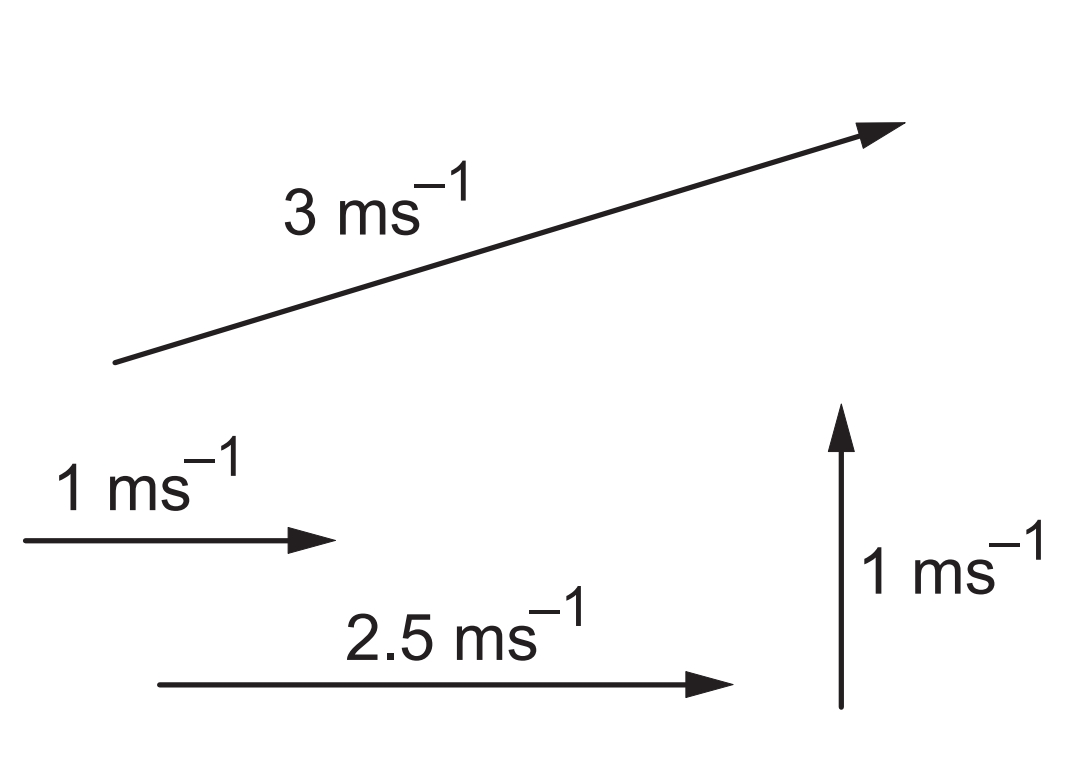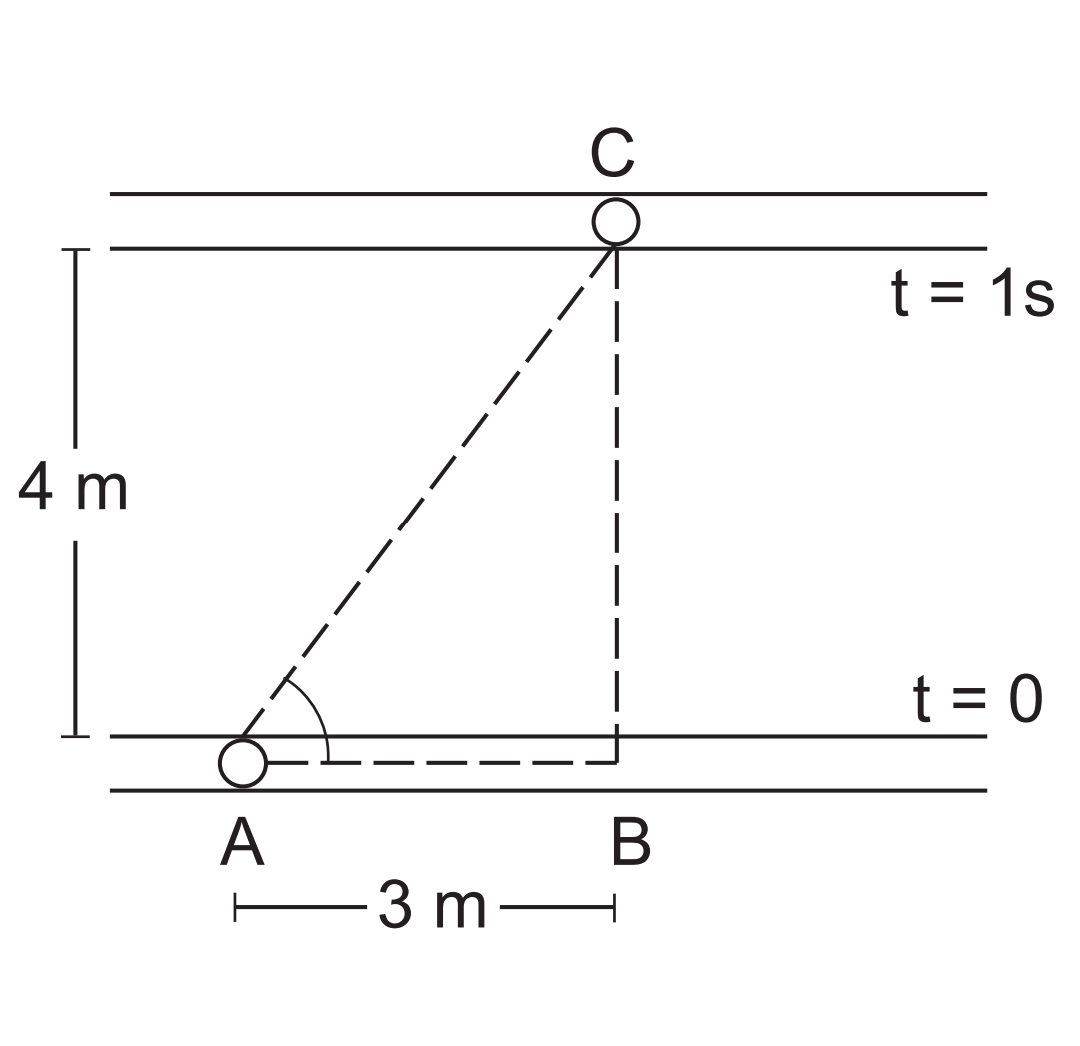# SCALAR QUANTITIES in physics {definition, examples, formula, explanation}

## Scalar Quantity Definitions:

• Scalar quantities are those physical quantities that have magnitude only and no direction.
• A Scalar quantity is that has size only and needs no other information to describe it.
• A Scalar quantity is a physical quantity that does not depend on the direction.

## Explanation of Scalar quantities.

Certain physical quantities are completely explained by a mathematical value (numerical value) and are derived according to the simples rules of the algebra.

let understand it by an example -

The mass of a system is described as that it is 10 kg. if two bodies have mass 10 kg and 5 kg respectively are added together to make a composite system, then the total mass of that system is the sum of their masses. i.e. 10 kg + 5 kg = 15 kg. these kinds of quantities are called Scalar quantities.

To completely describe certain physical quantities there is a numerical value, as well as a direction, is required.

An example of such kind of physical quantity is VELOCITY. The magnitude of velocity is represented by a number such as 7 m/s. it tells that how fast the particle is moving. but the description of velocity becomes complete only when the direction of velocity is also specified.

let's understand it through the below figure:In the figure given above, the velocity is represented by drawing a parallel line and putting a line showing the direction of velocity. A particular length is decided that represents 1 m/s and the length of the line representing a velocity of 5 m/s may be taken as 5 times this unit length. The above figure shows representations of several velocities in this scheme. The front end is called the head and the rear end is called the tail.

Further, if a particle is given two velocities simultaneously its resultant velocity is different from the two velocities, and the resultant velocity is obtained by using a special rule.

Suppose a small spherical object is moving inside a long tube at a speed of 3 m/s and the tube itself is moving in the room at a speed of 4 m/s along a direction perpendicular to its length. Then in which direction and how fast the ball is moving when seen from the room?The above fig. shows the positions of the tube and the ball at t = 0 and t = 1 s (t = time). As by geometry, it shows that the ball has moved 5 m in a direction ∅ = 53° from the tube. So the resultant velocity of the ball is 5 m/s along the direction.

The common rule for finding the resultant of two velocities is as follow:

Draw a line AB representing the first velocity with B as the head. Draw another line BC representing the second velocity with its tail B coinciding with the head of the first line. The line AC with A as the tail and C as the head represents the resultant velocity.The above fig. shows the construction.

The resultant is also called the sum of the two velocities. We have added the two velocities AB and BC and have obtained the sum AC. This rule of addition is called the "TRIANGLE RULE OF ADDITION".

## Scalar quantity examples

here are some of the important examples of Scalar quantities-
Mass (kg)
Speed (distance/time)
Volume (cubic meter)
Work (Force×displacement)
Time (second)
Power (work/time)
Energy (work/per unit time).

## Scalar quantity formula

Two scalar quantities form a derived Scalar Quantity i.e. a mathematical formula by multiplying or divide with each other. For example, Speed is a derived scalar quantity generated by dividing distance with time. where both distance and time are also scalar quantities

## Scalar quantity and Vector quantity difference

The difference between scalar quantity and vector quantity is that a Scalar quantity has magnitude only and no direction whereas a Vector quantity has both magnitude and direction as well as obey the Triangle Law.

The below table describes the clear difference between Scalar quantity and Vector quantity:

 Component Scalar Quantity Vector Quantity Magnitude Yes Yes Direction No Yes Triangle Law No Yes